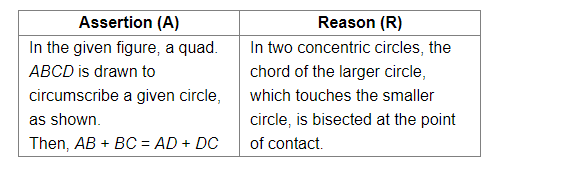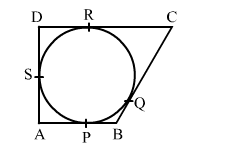# Solve this`
Question:Solution:

(d) Assertion(A) is false and Reasoning(R) is true.

Assertion: In this situation given in the diagram, the sum of opposite sides is always equal.
So, the correct relation should be: AB + CD = AD + CB
Hence, the assertion is false.
Reasoning: We know that in two concentric circles, the chord of the larger circle, which touches( or acts as a tangent to) the smaller circle, is bisected at the point of contact.
Therefore, Reasoning (R) is correct.
Hence, the correct answer is option (d).